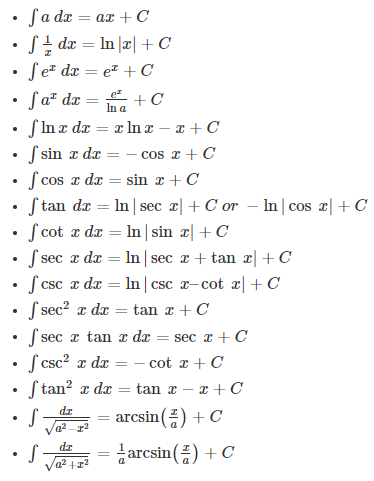# Integral Calculus

Integral Calculus is the branch of calculus where we study integrals and their properties. Integration is an essential concept which is the inverse process of differentiation. Both the integral and differential calculus are related to each other by the fundamental theorem of calculus. In this article, you will learn what is integral calculus, why it is used, its types, formulas, examples, and applications of integral calculus in detail.

## Integration Calculus

If we know the f’ of a function that is differentiable in its domain, we can then calculate f. In differential calculus, we used to call f’, the derivative of the function f. In integral calculus, we call f the anti-derivative or primitive of the function f’. And the process of finding the anti-derivatives is known as anti-differentiation or integration. As the name suggests, it is the inverse of finding differentiation.

### Types of Integrals

Integration can be classified into two different categories, namely,

• Definite Integral
• Indefinite Integral

Definite Integral

An integral that contains the upper and lower limits (i.e.) start and end value is known as a definite integral. The value of x is restricted to lie on a real line, and a definite Integral is also called a Riemann Integral when it is bound to lie on the real line.

A definite Integral is represented as:

$$\begin{array}{l}\int_{a}^{b}f(x)dx\end{array}$$

Indefinite Integral

Indefinite integrals are not defined using the upper and lower limits. The indefinite integrals represent the family of the given function whose derivatives are f, and it returns a function of the independent variable.

The integration of a function f(x) is given by F(x) and it is represented by:

∫f(x) dx = F(x) + C

where R.H.S. of the equation means integral off(x) with respect to x

F(x) is called anti-derivative or primitive.

f(x) is called the integrand.

dx is called the integrating agent.

C is called the constant of integration.

x is the variable of integration.

It may seem strange that there exist an infinite number of anti-derivatives for a function f. Taking an example will clarify it. Let us take f’ (x) = 3x2. By hit and trial, we can find out that its anti-derivative is F(x) = x3. This is because if you differentiate F with respect to x, you will get 3x2. There is only one function that we got as the anti-derivative of f. Let us now differentiate G(x)= x+ 9 with respect to x. Again we would get the same derivative, i.e. f. This gives us an important insight. Since the differentiation of all the constants is zero, we can write any constant with x3, and the derivative would still be equal to f. So, there are infinite constants that can be substituted for c in the equation F(x) = x+ C. Hence, there are infinite functions whose derivative is equal to f. And hence, there are infinite functions whose derivative is equal to 3x2. C is called an arbitrary constant. It is sometimes also referred to as the constant of integration.

## Integral Calculus Formulas

Similar to differentiation formulas, we have integral formulas as well. Let us go ahead and look at some of the integral calculus formulas.### Methods of Finding Integrals of Functions

We have different methods to find the integral of a given function in integral calculus. The most commonly used methods of integration are:

It is also possible to integrate the given function using the partial fractions technique.

## Uses of Integral Calculus

Integral Calculus is mainly used for the following two purposes:

1. To calculate f from f’. If a function f is differentiable in the interval of consideration, then f’ is defined. In differential calculus, we have already seen how to calculate derivatives of a function, and we can “undo” that with the help of integral calculus.
2. To calculate the area under a curve.

Until now, we have learned that areas are always positive. But as a matter of fact, there is something called a signed area.

## Video Lesson

### Integral Calculus## Application of Integral Calculus

The important applications of integral calculus are as follows. Integration is applied to find:

• The area between two curves
• Centre of mass
• Kinetic energy
• Surface area
• Work
• Distance, velocity and acceleration
• The average value of a function
• Volume

### Integral Calculus Examples

Below are the Integral Calculus Problems and Solutions.

Example 1: Find the integral of the function f(x) = √x.

Solution:

Given,

f(x) = √x

∫f(x) dx = ∫√x dx

$$\begin{array}{l}\int \sqrt{x}\ dx = \int x^{\frac{1}{2}}\ dx\end{array}$$

We know that,

$$\begin{array}{l}\int x^{n}\ dx = \frac{x^{n+1}}{n+1}+C\end{array}$$

Now,

$$\begin{array}{l}\int \sqrt{x}\ dx = \frac{x^{\frac{1}{2}+1}}{\frac{1}{2}+1}+C\\= \frac{x^{\frac{1+2}{2}}}{\frac{1+2}{2}}+C\\=\frac{2}{3}x^{\frac{3}{2}}+C\end{array}$$

Example 2: Find the integral of cos2n with respect to n.

Solution:

Let f(n) = cos2

we know that 2 cos2A = cos 2A + 1

So, f(n) = (1/2)(cos 2n + 1)

Let us find the integral of f(n).

∫f(n) dn = ∫(1/2)(cos 2n + 1) dn

= (1/2) ∫(cos 2n + 1) dn

= (1/2) ∫cos 2n dn + (1/2)∫1 dn

= (1/2) (sin 2n/2) + (1/2) n + C

= (sin 2n/4) + (n/4) + C

(1/4)[sin 2n + n] + C

Example 3: Evaluate:

$$\begin{array}{l}\int_{0}^{\pi}sin x\ dx\end{array}$$

Solution:

We know that,

∫sin x dx = cos x  + C

$$\begin{array}{l}\int_{0}^{\pi}sin x\ dx = [-cosx]_{0}^{\pi}\end{array}$$

= -cos π – (-cos 0)

= -(-1) + 1

= 1 + 1

= 2

## Video Lesson on Class 12 Important Calculus QuestionsRegister with BYJU’S – The Learning App and download the integral calculus pdf to learn the formulas and examples.